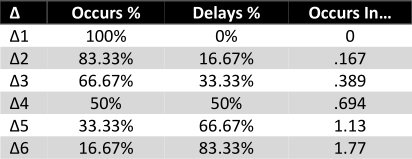### Risk Dice vs Deltas

First, I want to give credit to dieheart's blog post which compares The Black Hack's usage dice to those of Macchiato Monsters. It sparked my interest in fun dice mechanics. :)

## As Resource Counters

In Macchiato Monsters Zero, you roll a funny shaped die representing a risk or a resource. If you roll 1-3, you have to decrease the size of the die. This represents a resource being depleted, or a risk becoming more likely. When you roll 1-3 on a d4, the resource is completely used up.

Deltas from Macchiato Micro are a remix of risk dice: instead of keeping track of a certain die size, you only have to use a d6 and keep track of a certain number to roll under. This is the 'delta' number Δ. When you roll d6 < Δ, the resource is depleted (or the risk becomes more likely) and Δ is decreased by 1.

If we were to swap the conditions of the Δ system so that d6 ≥ Δ means depletion, the chances resemble something much closer to the risk die system. This would mean that a Δ2 item has about 1 use at best! Relative to the risk die system, though, the number of uses at each level is generally lower.

By comparing this table with the risk dice, we can see that unlike the risk die system where higher rank means lower chance of depletion, the higher the Δ, the greater chance of depletion! This means that a low-ranking Δ2 item has almost multiple guaranteed uses, and a higher-ranking Δ4 item is still only a 45% improvement over Δ2.

In any case, I think this is a really cool and useful way of handling things and Δ is such a good notation! I can see this being super useful as a replacement for clocks or HP too :) And I prefer it to usage dice if only because I'm prejudiced against all weird 3D solids except for dodecahedrons.

Update: New Delta Mechanic

The latest update has it so that the Δ changes to the number rolled < Δ, not to Δ - 1. This changes how many total uses (i.e. depletion misses) there are at each level, since we're not counting from the next lowest Δ but from the average of what you might roll next.

This results in the number of total uses flattening at Δ3. Keep in mind, though, that at each step the risk of danger increases (if Δ represents a risk and not a resource). So by the nature of that, it doesn't seem to work well for resource management. The table also doesn't accurately represent rolling ≥ Δ as a failure state where the "number of uses" terminates (because the avalanche finally happens, for example). This is a really important distinction between risks and resources: you want to roll high for resources to keep them, and roll low for risks to avoid them. So, it might work well to have Δ := Δ - 1 (subtract 1) for resources, and Δ := d6 < Δ (change to roll lower than current Δ) for risks.

Update 2: The part below is incorrect! I thought that the risk occurs when you roll equal/over delta, but that’s not the case—it only occurs when delta 2 is downstepped. Therefore the graphs above work for risks too!

## As Risk Counters (INCORRECT)

Using these mechanics as risk counters result in very different results than using them as resource counters, for the simple reason that what counts as a 'success' on the resource die (rolling to avoid depleting the resource) counts as a 'failure' on the risk die (the disaster strikes).

This is where the delta dice shine: because you're more likely to roll d6 ≥ Δ as Δ → 1, this means that the risk becomes more likely as time goes on. I can't speak to how this mechanic works in Macchiato Monsters with risk dice, but the opposite is true here if it works the same way as in Micro: as the risk die is downgraded, the chance of depletion and risk non-occurrence increases.

Here is the chart for how I am certain Micro ends up, assuming that after each delay Δ := Δ - 1. When you roll the Δ die, there is a (Δ-1)/6 chance that the risk is delayed and Δ decreases, and there is a (7 -Δ)/6 chance that the risk occurs. When the risk is delayed, this means we have one extra turn to breathe before rolling again with worse chances.At Δ2, the risk will either occur immediately (83%) or it will occur immediately on the next turn (17%) because it has become Δ1. We represent this as 0*.8333 + 1*.1667 = .1667.

At Δ3, the risk will either occur immediately (67%) or it will delay until the next turn where it is 17% likely (33%). This means it will either occur now, or it will occur in 1+.1667 turns. We represent this as 0*.6667 + 1*(1+1.1667) = .389.

At Δ4, the risk will either occur immediately (50%) or it will delay until the next turn where it is 39% likely (50%). This means it will occur in 0*.5 + 1*(1+.389) = .694 turns.

When the number of turns > 1, this means it's more likely that the risk will delay than occur.

If Micro is analogous to Monsters, this is how Monsters ends up using the same rationale:

We can see here how the lower the risk die, the longer it will take for the risk to actually occur. This is because as the delay % increases, the occurrence % decreases at the same rate.

Let's go back to Micro, using the different rule that when d6 < Δ, Δ := d6 instead of Δ := Δ - 1.

This results in overall more frequent occurrences. Keep in mind that the outcome of replacing Δ with d6 is that it could become Δ1, resulting in an immediate risk occurrence on the next turn. This drastically increases the frequency at which the risk will actually occur.

1.Thank you!! Sorry it took me a while to catch this :)